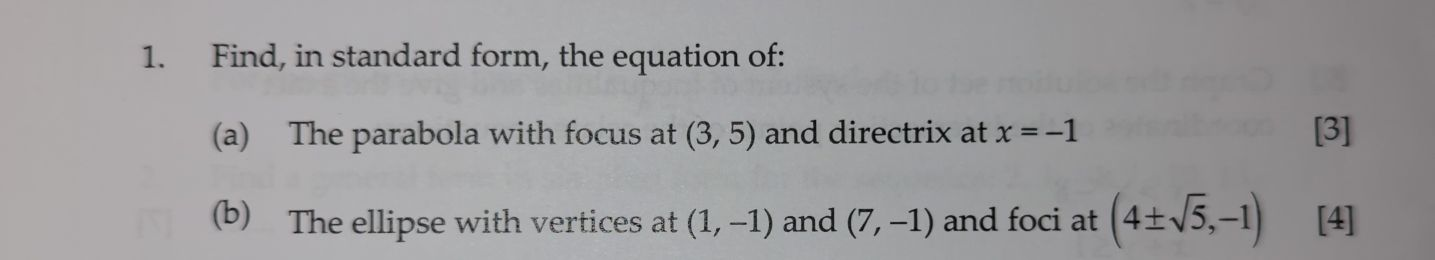### ¿Todavía tienes preguntas de matemáticas?

Pregunte a nuestros tutores expertos
Algebra
Pregunta1. Find, in standard form, the equation of:

(a) The parabola with focus at $$( 3,5 )$$ and directrix at $$x = - 1$$

(b) The ellipse with vertices at $$( 1 , - 1 )$$ and $$( 7 , - 1 )$$ and foci at $$( 4 \pm \sqrt { 5 } , - 1 )$$

(a)

$$p= 3- (- 1)= 4\\2p= 8$$

Center: $$(\frac{3- 1}{2},5)= (1,5)$$

$$(y- 5)^{2} = 8(x- 1)$$

(b)

$$2a= 7- 1= 6\Rightarrow a= 3\\c= 4+ \sqrt{5} - \frac{7+ 1}{2}= \sqrt{5} \\b= \sqrt{3^{2}- 5 } = 2$$

Center: $$(\frac{7+ 1}{2},- 1)= (4,- 1)$$

$$\frac{(x- 4)^{2} }{9} + \frac{(y+ 1)^{2} }{4} = 1$$

Solución
View full explanation on CameraMath App.